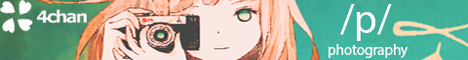[a / b / c / d / e / f / g / gif / h / hr / k / m / o / p / r / s / t / u / v / vg / vm / vmg / vr / vrpg / vst / w / wg] [i / ic] [r9k / s4s / vip / qa] [cm / hm / lgbt / y] [3 / aco / adv / an / bant / biz / cgl / ck / co / diy / fa / fit / gd / hc / his / int / jp / lit / mlp / mu / n / news / out / po / pol / pw / qst / sci / soc / sp / tg / toy / trv / tv / vp / vt / wsg / wsr / x / xs] [Settings] [Search] [Mobile] [Home]
Board
/wsr/ - Worksafe RequestsSo I turned it into regular rectangular form equation but the graph isn't coming right. I'll be attaching my conversion, the graph i got and the actual graph.
>>
>>
The Graph from the reduction
>>
Actual Graph
>>
>>1420002
Hard to follow, but I noticed on the first line
> cos 2θ = √((1+cos θ)/2)
which is backwards. The correct formula is
> cos(θ/2) = √((1+cos θ)/2)
>>
>>1420012
(continued)

So the correct formula is
r = cos(2θ) = 2cos^2(θ) - 1
with x = r cos(θ) we get
r = 2(x/r)^2 - 1
multiplying both sides by r^2:
r^3 = 2x^2 - r^2
r^3 + r^2 = 2x^2
x = √((r^3 + r^2)/2) = r√((1+r^2) / 2)
>>
>>1420014
(continued)

from
x^2 = (r^3 + r^2) / 2
we get
x^2 = ((x^2 + y^2)^(3/2) + (x^2 + y^2)) / 2

I only get half of the expected plot. I think this is because squaring gets rid of some minus signs. I have to think about it.
>>
>>1420016
(continued)

A minus sign gives the other half. I'm not sure how to get them both in Desmos.
>>
(continued)

So the plot from your textbook doesn't restrict r to positive values only. The vertical leaves of the rose correspond to parts where r=cos(2θ) is negative.

So we have
r = ±√(x^2 + y^2)
The minus sign disappears in the term r^2 but not in the term r^3.
>>
>>1420012
WHAT THE FUCK, I need to sleep. Thank you very much brother.
>>
>>1420017
There are tabs to write below where you wrote the top equation.
>>
>>1420002
you know you can plot polar plots in desmos right? just use r and theta
>>
>>1420030
Didn't know that, but the point is to practice conversion between polar and rectangular coordinates and check his answer.
>>
>>1420020
How can Root x2 + y2 be negative, sum of two squares will always be positive.
>>
>>1420262
it wont be
but the solution to a^2 = b is +-sqrt(a) = b
>>
>>1420263
other way around
+-sqrt(b) = a
>>
>>1420263
Yep i got it, i am a dumb fuck. In case of variables there's always plus and minus case.
>>
>>1420005
What book?
>>
>>1420270
looks like Stewart Calculus, but OP seems to have a different edition
>>
>>1420643
OP here,

It's from Pre-Calculus by Stalwart, Chapter - 8

Delete Post: [File Only] Style: